Anúncio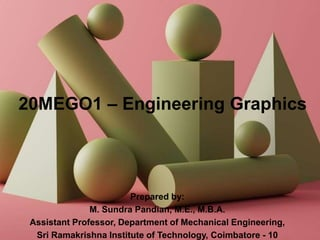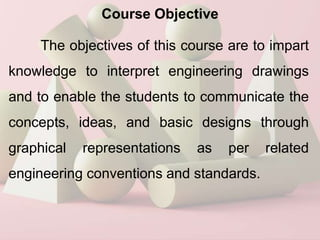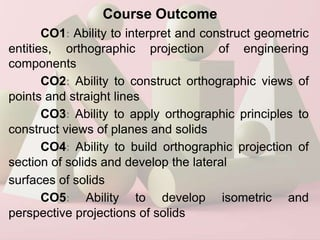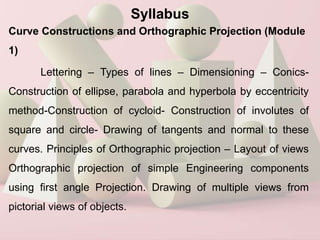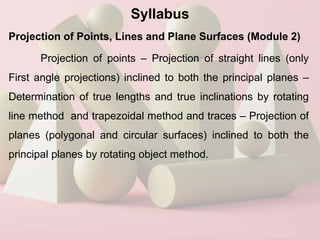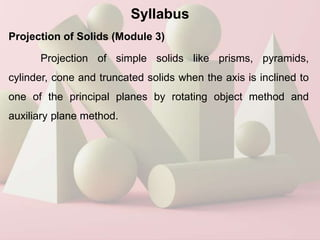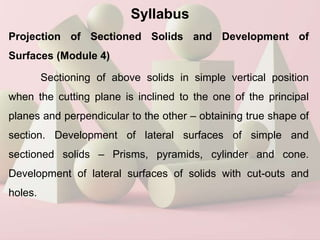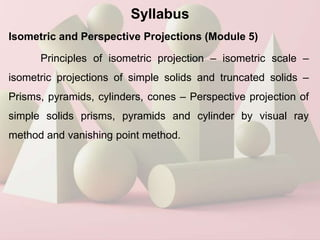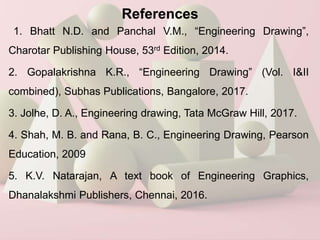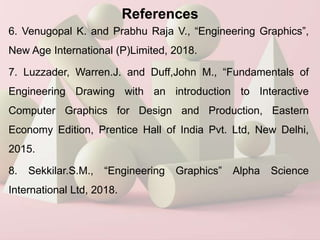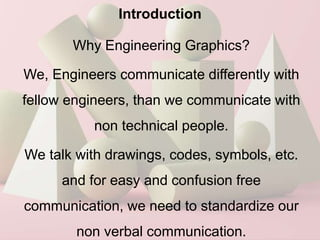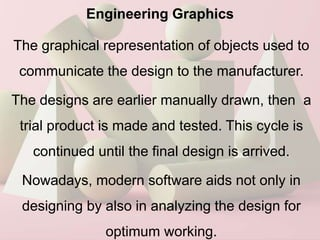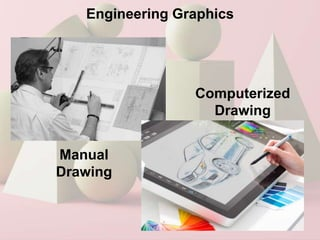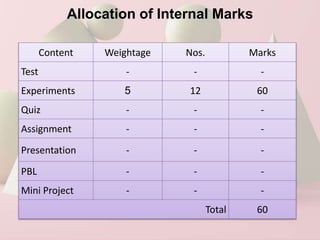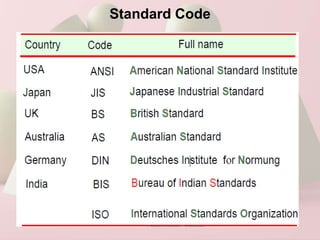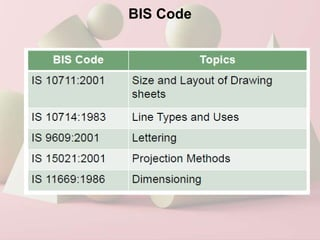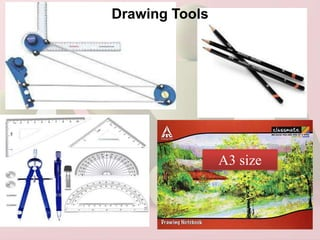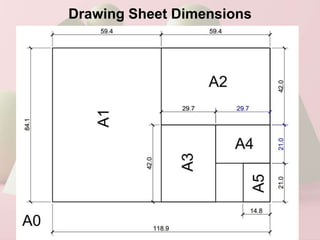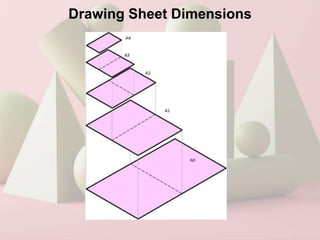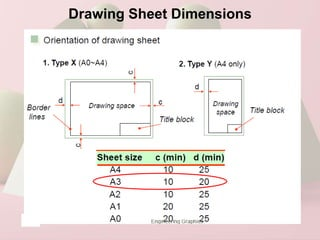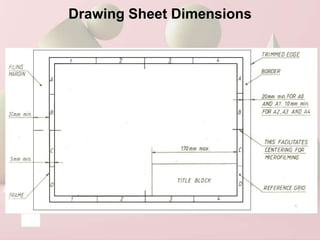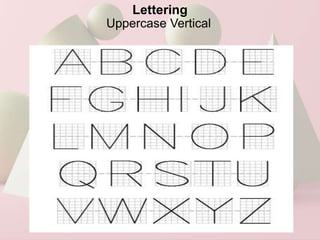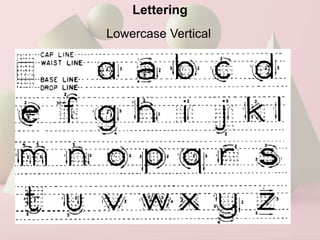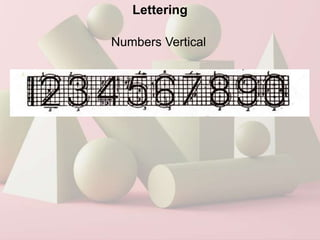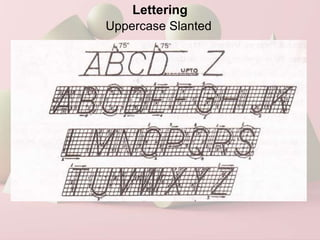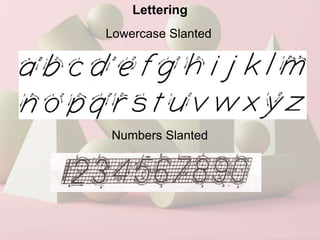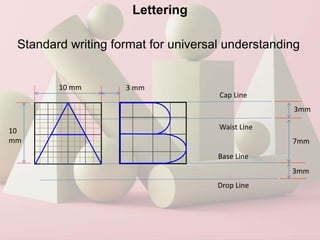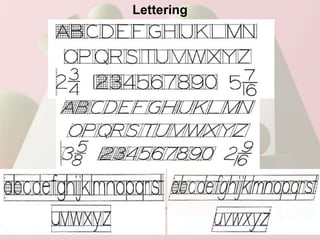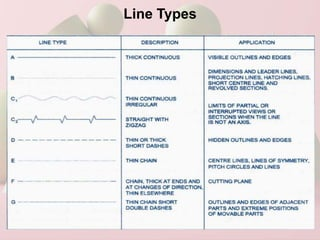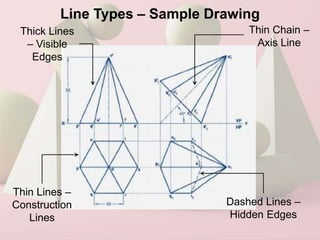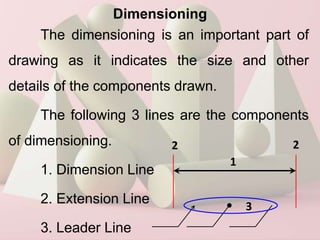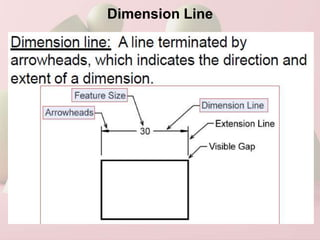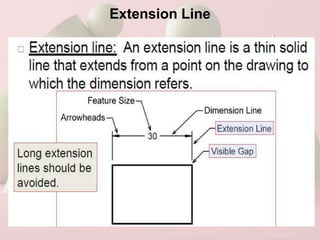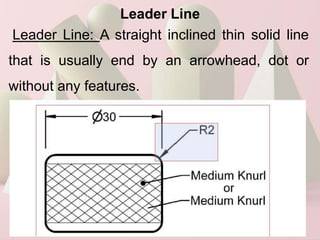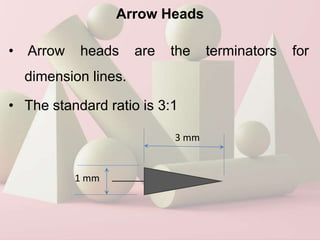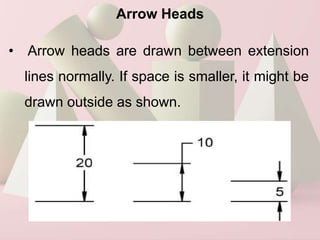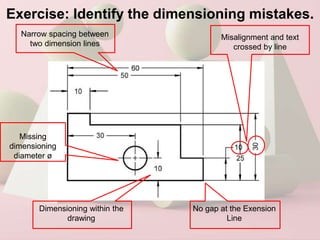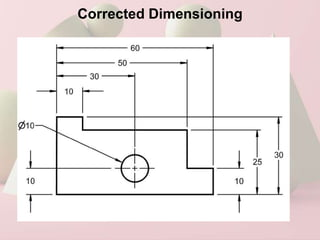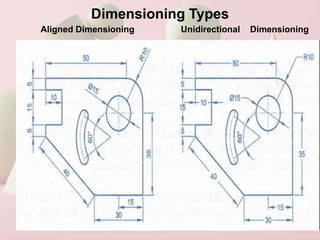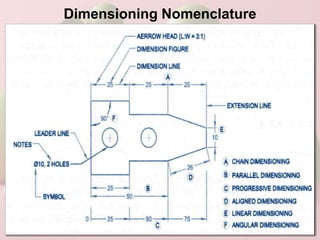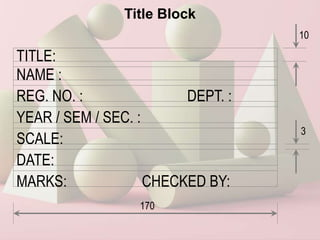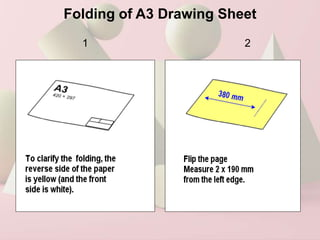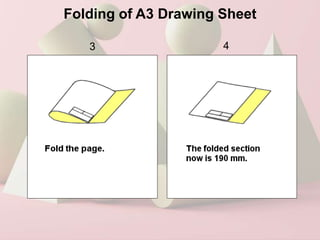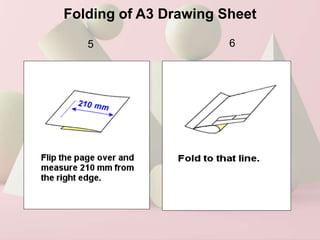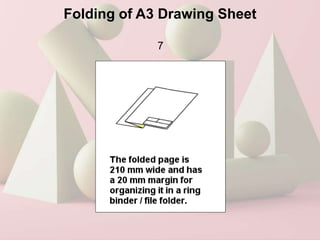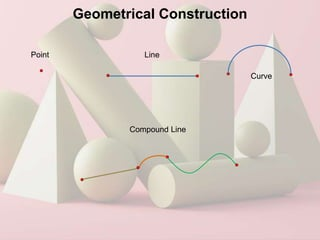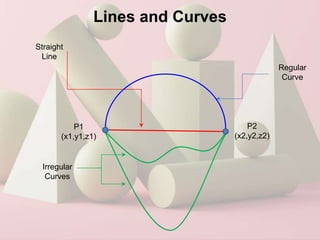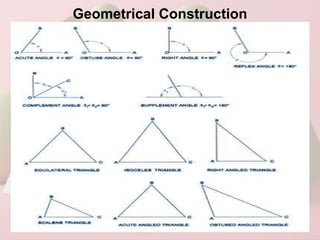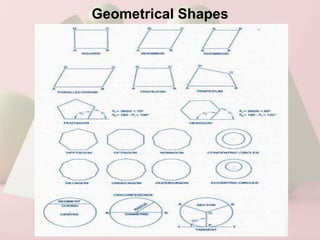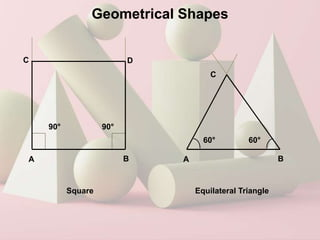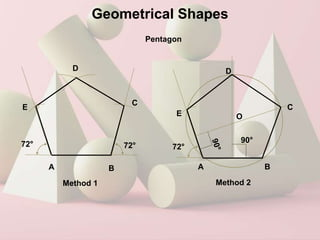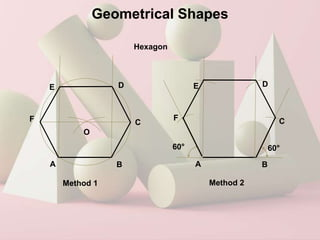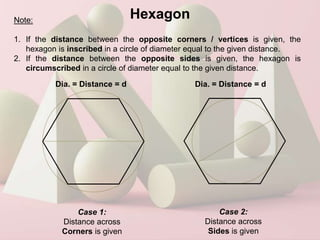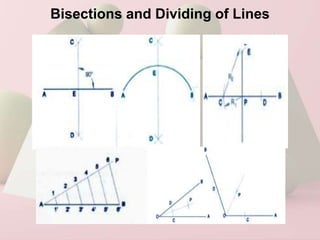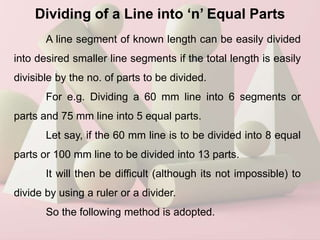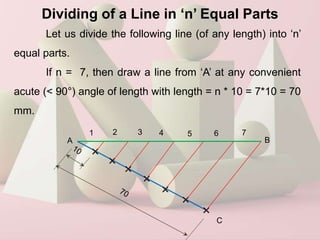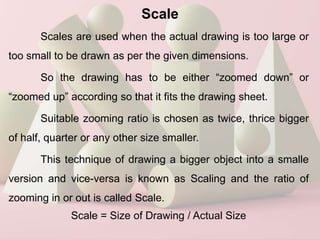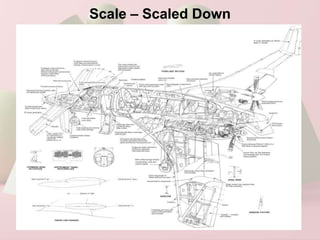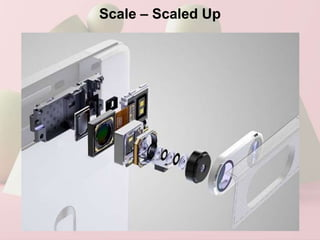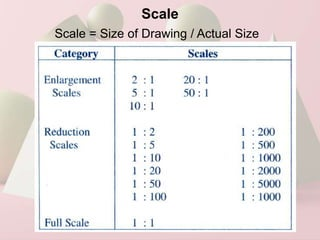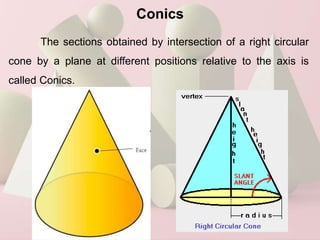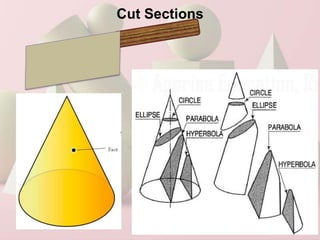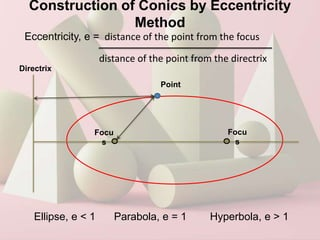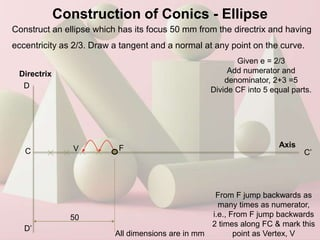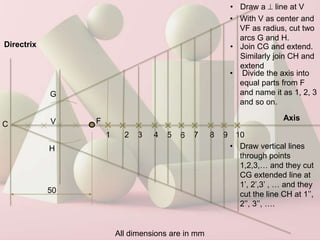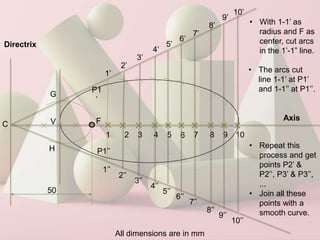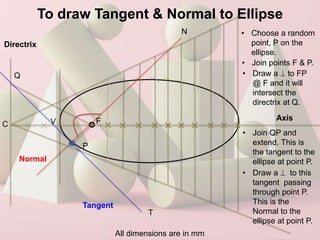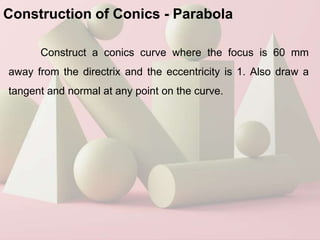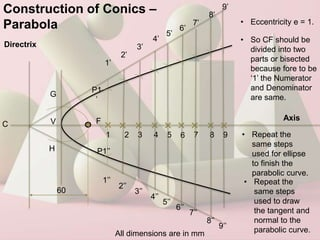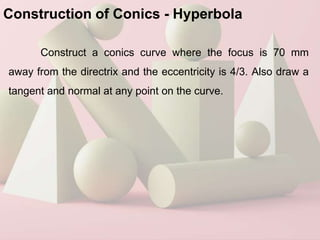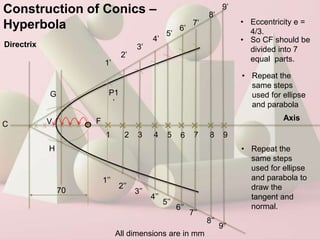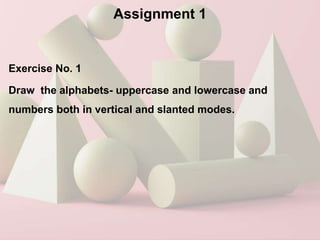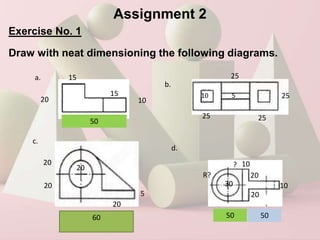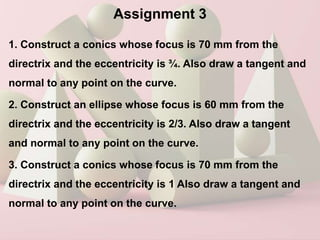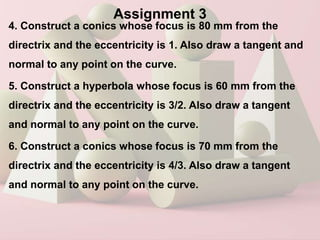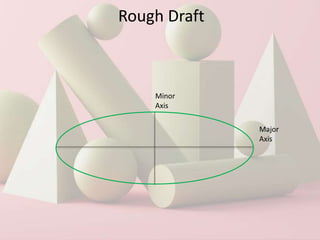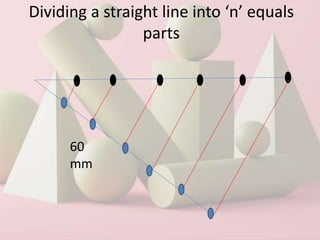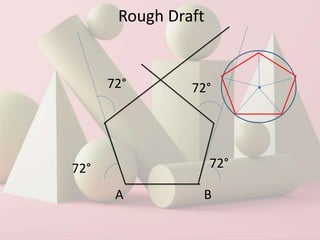1 de 79
Anúncio

### Eg unit 1 1

1. 20MEGO1 – Engineering Graphics Prepared by: M. Sundra Pandian, M.E., M.B.A. Assistant Professor, Department of Mechanical Engineering, Sri Ramakrishna Institute of Technology, Coimbatore - 10
2. Course Objective The objectives of this course are to impart knowledge to interpret engineering drawings and to enable the students to communicate the concepts, ideas, and basic designs through graphical representations as per related engineering conventions and standards.
3. Course Outcome CO1: Ability to interpret and construct geometric entities, orthographic projection of engineering components CO2: Ability to construct orthographic views of points and straight lines CO3: Ability to apply orthographic principles to construct views of planes and solids CO4: Ability to build orthographic projection of section of solids and develop the lateral surfaces of solids CO5: Ability to develop isometric and perspective projections of solids
4. Syllabus Curve Constructions and Orthographic Projection (Module 1) Lettering – Types of lines – Dimensioning – Conics- Construction of ellipse, parabola and hyperbola by eccentricity method-Construction of cycloid- Construction of involutes of square and circle- Drawing of tangents and normal to these curves. Principles of Orthographic projection – Layout of views Orthographic projection of simple Engineering components using first angle Projection. Drawing of multiple views from pictorial views of objects.
5. Syllabus Projection of Points, Lines and Plane Surfaces (Module 2) Projection of points – Projection of straight lines (only First angle projections) inclined to both the principal planes – Determination of true lengths and true inclinations by rotating line method and trapezoidal method and traces – Projection of planes (polygonal and circular surfaces) inclined to both the principal planes by rotating object method.
6. Syllabus Projection of Solids (Module 3) Projection of simple solids like prisms, pyramids, cylinder, cone and truncated solids when the axis is inclined to one of the principal planes by rotating object method and auxiliary plane method.
7. Syllabus Projection of Sectioned Solids and Development of Surfaces (Module 4) Sectioning of above solids in simple vertical position when the cutting plane is inclined to the one of the principal planes and perpendicular to the other – obtaining true shape of section. Development of lateral surfaces of simple and sectioned solids – Prisms, pyramids, cylinder and cone. Development of lateral surfaces of solids with cut-outs and holes.
8. Syllabus Isometric and Perspective Projections (Module 5) Principles of isometric projection – isometric scale – isometric projections of simple solids and truncated solids – Prisms, pyramids, cylinders, cones – Perspective projection of simple solids prisms, pyramids and cylinder by visual ray method and vanishing point method.
9. References 1. Bhatt N.D. and Panchal V.M., “Engineering Drawing”, Charotar Publishing House, 53rd Edition, 2014. 2. Gopalakrishna K.R., “Engineering Drawing” (Vol. I&II combined), Subhas Publications, Bangalore, 2017. 3. Jolhe, D. A., Engineering drawing, Tata McGraw Hill, 2017. 4. Shah, M. B. and Rana, B. C., Engineering Drawing, Pearson Education, 2009 5. K.V. Natarajan, A text book of Engineering Graphics, Dhanalakshmi Publishers, Chennai, 2016.
10. References 6. Venugopal K. and Prabhu Raja V., “Engineering Graphics”, New Age International (P)Limited, 2018. 7. Luzzader, Warren.J. and Duff,John M., “Fundamentals of Engineering Drawing with an introduction to Interactive Computer Graphics for Design and Production, Eastern Economy Edition, Prentice Hall of India Pvt. Ltd, New Delhi, 2015. 8. Sekkilar.S.M., “Engineering Graphics” Alpha Science International Ltd, 2018.
11. Introduction Why Engineering Graphics? We, Engineers communicate differently with fellow engineers, than we communicate with non technical people. We talk with drawings, codes, symbols, etc. and for easy and confusion free communication, we need to standardize our non verbal communication.
12. Engineering Graphics The graphical representation of objects used to communicate the design to the manufacturer. The designs are earlier manually drawn, then a trial product is made and tested. This cycle is continued until the final design is arrived. Nowadays, modern software aids not only in designing by also in analyzing the design for optimum working.
13. Engineering Graphics Manual Drawing Computerized Drawing
14. Allocation of Internal Marks Content Weightage Nos. Marks Test - - - Experiments 5 12 60 Quiz - - - Assignment - - - Presentation - - - PBL - - - Mini Project - - - Total 60
15. Standard Code
16. BIS Code
17. Drawing Tools A3 size
18. Drawing Sheet Dimensions
19. Drawing Sheet Dimensions
20. Drawing Sheet Dimensions
21. Drawing Sheet Dimensions
22. Lettering Uppercase Vertical
23. Lettering Lowercase Vertical
24. Lettering Numbers Vertical
25. Lettering Uppercase Slanted
26. Lettering Lowercase Slanted Numbers Slanted
27. Lettering Standard writing format for universal understanding 3mm 3mm 7mm 10 mm 10 mm 3 mm Cap Line Waist Line Base Line Drop Line
28. Lettering
29. Line Types
30. Line Types – Sample Drawing Thick Lines – Visible Edges Thin Lines – Construction Lines Dashed Lines – Hidden Edges Thin Chain – Axis Line
31. Dimensioning The dimensioning is an important part of drawing as it indicates the size and other details of the components drawn. The following 3 lines are the components of dimensioning. 1. Dimension Line 2. Extension Line 3. Leader Line 1 2 3 2
32. Dimension Line
33. Extension Line
34. Leader Line Leader Line: A straight inclined thin solid line that is usually end by an arrowhead, dot or without any features.
35. Arrow Heads • Arrow heads are the terminators for dimension lines. • The standard ratio is 3:1 3 mm 1 mm
36. Arrow Heads • Arrow heads are drawn between extension lines normally. If space is smaller, it might be drawn outside as shown.
37. Exercise: Identify the dimensioning mistakes. Narrow spacing between two dimension lines Dimensioning within the drawing Missing dimensioning diameter ø No gap at the Exension Line Misalignment and text crossed by line
38. Corrected Dimensioning
39. Dimensioning Types Aligned Dimensioning Unidirectional Dimensioning
40. Dimensioning Nomenclature
41. Title Block NAME : REG. NO. : YEAR / SEM / SEC. : DEPT. : TITLE: SCALE: DATE: MARKS: CHECKED BY: 170 3 10
42. Folding of A3 Drawing Sheet 1 2
43. Folding of A3 Drawing Sheet 4 3
44. Folding of A3 Drawing Sheet 5 6
45. Folding of A3 Drawing Sheet 7
46. Geometrical Construction Point Line Curve Compound Line
47. Lines and Curves P1 (x1,y1,z1) P2 (x2,y2,z2) Straight Line Regular Curve Irregular Curves
48. Geometrical Construction
49. Geometrical Shapes
50. Geometrical Shapes Square 90° 90° A B C D Equilateral Triangle A B C 60° 60°
51. Geometrical Shapes Pentagon A B 72° 72° Method 1 Method 2 A B 72° E E D C 90° O C D
52. D C Geometrical Shapes Hexagon A B O C D E F A B 60° 60° E F Method 1 Method 2
53. Hexagon Note: 1. If the distance between the opposite corners / vertices is given, the hexagon is inscribed in a circle of diameter equal to the given distance. 2. If the distance between the opposite sides is given, the hexagon is circumscribed in a circle of diameter equal to the given distance. Case 1: Distance across Corners is given Dia. = Distance = d Case 2: Distance across Sides is given Dia. = Distance = d
54. Bisections and Dividing of Lines
55. Dividing of a Line into ‘n’ Equal Parts A line segment of known length can be easily divided into desired smaller line segments if the total length is easily divisible by the no. of parts to be divided. For e.g. Dividing a 60 mm line into 6 segments or parts and 75 mm line into 5 equal parts. Let say, if the 60 mm line is to be divided into 8 equal parts or 100 mm line to be divided into 13 parts. It will then be difficult (although its not impossible) to divide by using a ruler or a divider. So the following method is adopted.
56. Let us divide the following line (of any length) into ‘n’ equal parts. If n = 7, then draw a line from ‘A’ at any convenient acute (< 90°) angle of length with length = n * 10 = 7*10 = 70 mm. Dividing of a Line in ‘n’ Equal Parts A B 1 6 7 4 5 2 3 C
57. Scale Scales are used when the actual drawing is too large or too small to be drawn as per the given dimensions. So the drawing has to be either “zoomed down” or “zoomed up” according so that it fits the drawing sheet. Suitable zooming ratio is chosen as twice, thrice bigger of half, quarter or any other size smaller. This technique of drawing a bigger object into a smalle version and vice-versa is known as Scaling and the ratio of zooming in or out is called Scale. Scale = Size of Drawing / Actual Size
58. Scale – Scaled Down
59. Scale – Scaled Up
60. Scale Scale = Size of Drawing / Actual Size
61. Conics The sections obtained by intersection of a right circular cone by a plane at different positions relative to the axis is called Conics.
62. Cut Sections
63. Construction of Conics by Eccentricity Method Eccentricity, e = distance of the point from the focus distance of the point from the directrix Focu s Focu s Point Directrix Ellipse, e < 1 Parabola, e = 1 Hyperbola, e > 1
64. Construction of Conics - Ellipse Construct an ellipse which has its focus 50 mm from the directrix and having eccentricity as 2/3. Draw a tangent and a normal at any point on the curve. F Directrix 50 All dimensions are in mm Given e = 2/3 Add numerator and denominator, 2+3 =5 Divide CF into 5 equal parts. C From F jump backwards as many times as numerator, i.e., From F jump backwards 2 times along FC & mark this point as Vertex, V V Axis D’ D C’
65. F Directrix 50 All dimensions are in mm C V • Draw a  line at V • With V as center and VF as radius, cut two arcs G and H. G H • Join CG and extend. Similarly join CH and extend Axis • Divide the axis into equal parts from F and name it as 1, 2, 3 and so on. 1 2 3 4 5 6 7 8 9 10 • Draw vertical lines through points 1,2,3,… and they cut CG extended line at 1’, 2’,3’ , … and they cut the line CH at 1’’, 2’’, 3’’, ….
66. F Directrix 50 All dimensions are in mm C V • With 1-1’ as radius and F as center, cut arcs in the 1’-1” line. G H Axis 1 2 3 4 5 6 7 8 9 10 1’ 2’ 3’ 4’ 5’ 6’ 7’ 8’ 9’ 10’ 1’’ 2’’ 3’’ 4’’ 5’’ 6’’ 7’’ 8’’ 9’’ 10’’ • The arcs cut line 1-1’ at P1’ and 1-1’’ at P1’’. P1 ’ P1’’ • Repeat this process and get points P2’ & P2’’, P3’ & P3’’, ... • Join all these points with a smooth curve.
67. To draw Tangent & Normal to Ellipse F Directrix All dimensions are in mm C V Axis • Choose a random point, P on the ellipse. P • Join points F & P. • Draw a  to FP @ F and it will intersect the directrix at Q. Q • Join QP and extend. This is the tangent to the ellipse at point P. Tangent • Draw a  to this tangent passing through point P. This is the Normal to the ellipse at point P. Normal N T
68. Construction of Conics - Parabola Construct a conics curve where the focus is 60 mm away from the directrix and the eccentricity is 1. Also draw a tangent and normal at any point on the curve.
69. Construction of Conics – Parabola F Directrix 60 All dimensions are in mm C V • Eccentricity e = 1. G H Axis 1 2 3 4 5 6 7 8 9 1’ 2’ 3’ 4’ 5’ 6’ 7’ 8’ 9’ 1’’ 2’’ 3’’ 4’’ 5’’ 6’’ 7’’ 8’’ 9’’ • So CF should be divided into two parts or bisected because fore to be ‘1’ the Numerator and Denominator are same. P1 ’ P1’’ • Repeat the same steps used for ellipse to finish the parabolic curve. • Repeat the same steps used to draw the tangent and normal to the parabolic curve.
70. Construction of Conics - Hyperbola Construct a conics curve where the focus is 70 mm away from the directrix and the eccentricity is 4/3. Also draw a tangent and normal at any point on the curve.
71. Construction of Conics – Hyperbola F Directrix 70 All dimensions are in mm C V • Eccentricity e = 4/3. G H Axis 1 2 3 4 5 6 7 8 9 1’ 2’ 3’ 4’ 5’ 6’ 7’ 8’ 9’ 1’’ 2’’ 3’’ 4’’ 5’’ 6’’ 7’’ 8’’ 9’’ • So CF should be divided into 7 equal parts. P1 ’ • Repeat the same steps used for ellipse and parabola • Repeat the same steps used for ellipse and parabola to draw the tangent and normal.
72. Syllabus Curve Constructions and Orthographic Projection (Module 1) Lettering – Types of lines – Dimensioning – Conics- Construction of ellipse, parabola and hyperbola by eccentricity method-Construction of cycloid- Construction of involutes of square and circle- Drawing of tangents and normal to these curves. Principles of Orthographic projection – Layout of views Orthographic projection of simple Engineering components using first angle Projection. Drawing of multiple views from pictorial views of objects.
73. Assignment 1 Exercise No. 1 Draw the alphabets- uppercase and lowercase and numbers both in vertical and slanted modes.
74. Assignment 2 Exercise No. 1 Draw with neat dimensioning the following diagrams. 20 15 50 15 10 a. b. 25 25 25 25 5 10 c. 60 20 20 20 5 20 d. 30 10 20 20 10 50 R? ? 50
75. Assignment 3 1. Construct a conics whose focus is 70 mm from the directrix and the eccentricity is ¾. Also draw a tangent and normal to any point on the curve. 2. Construct an ellipse whose focus is 60 mm from the directrix and the eccentricity is 2/3. Also draw a tangent and normal to any point on the curve. 3. Construct a conics whose focus is 70 mm from the directrix and the eccentricity is 1 Also draw a tangent and normal to any point on the curve.
76. Assignment 3 4. Construct a conics whose focus is 80 mm from the directrix and the eccentricity is 1. Also draw a tangent and normal to any point on the curve. 5. Construct a hyperbola whose focus is 60 mm from the directrix and the eccentricity is 3/2. Also draw a tangent and normal to any point on the curve. 6. Construct a conics whose focus is 70 mm from the directrix and the eccentricity is 4/3. Also draw a tangent and normal to any point on the curve.
77. Rough Draft Major Axis Minor Axis
78. Dividing a straight line into ‘n’ equals parts 60 mm
79. Rough Draft A B 72° 72° 72° 72°
Anúncio